# Solving Simultaneous Equations Graphically (1)

In this worksheet, students solve simple simultaneous equations graphically by finding the point of intersection on an x-y graph.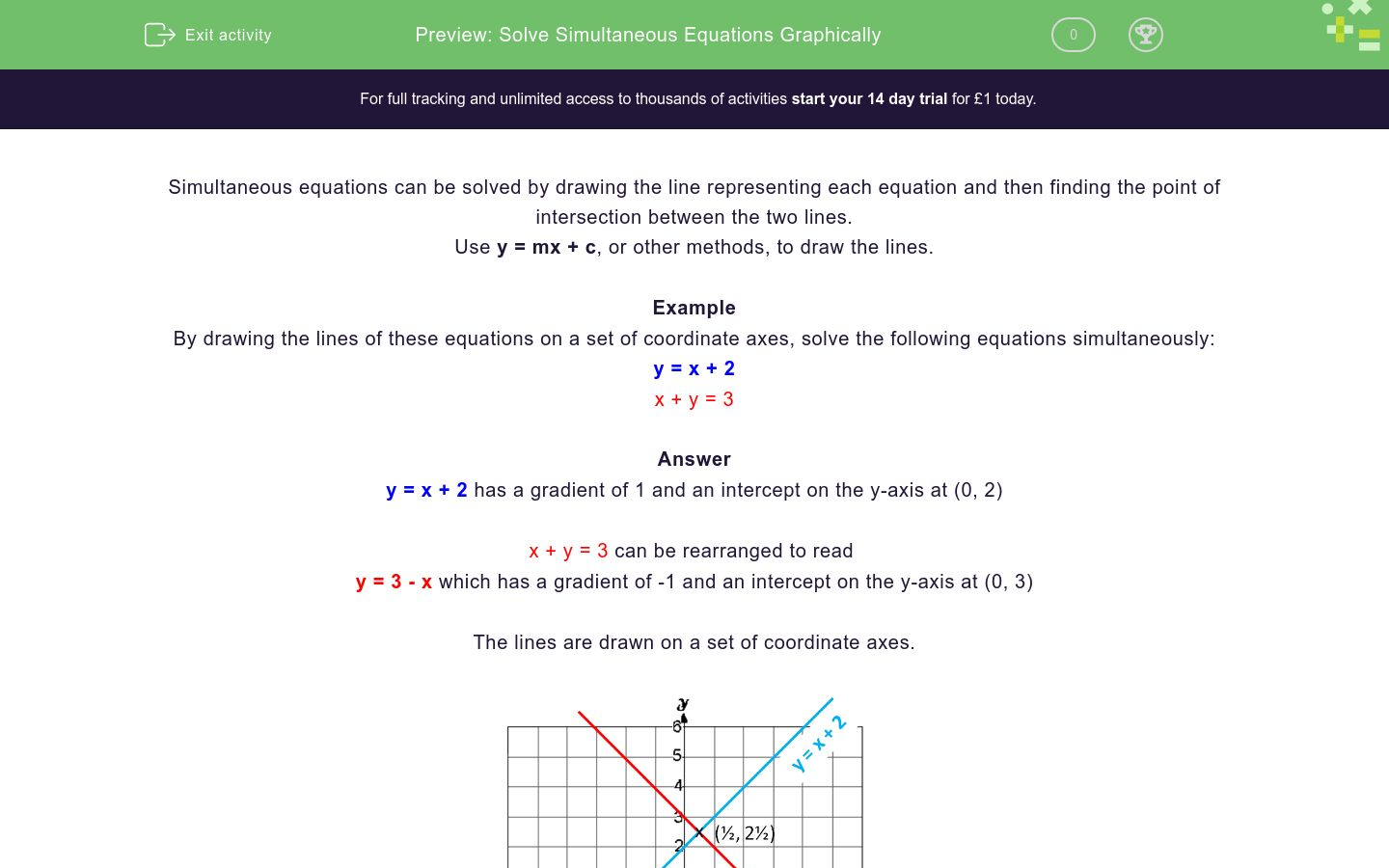Key stage:  KS 4

Curriculum topic:  Algebra

Difficulty level:### QUESTION 1 of 10

Simultaneous equations can be solved by drawing the line representing each equation and then finding the point of intersection between the two lines.

Use y = mx + c, or other methods, to draw the lines.

Example

By drawing the lines of these equations on a set of coordinate axes, solve the following equations simultaneously:

y = x + 2

x + y = 3

y = x + 2 has a gradient of 1 and an intercept on the y-axis at (0, 2)

x + y = 3 can be rearranged to read

y = 3 - x which has a gradient of -1 and an intercept on the y-axis at (0, 3)

The lines are drawn on a set of coordinate axesThe point of intersection is (½, 2½) and this is the only point at which both equations are satisfied.

So the solution to the simultaneous equations is

x = ½, y = 2½

By drawing the lines of these equations on a set of coordinate axes, solve the following equations simultaneously:

y = x - 2

y = 4 - x

x = 1, y = 2

x = 1, y = 3

x = 3, y = 1

By drawing the lines of these equations on a set of coordinate axes, solve the following equations simultaneously:

y = x - 2

y = 6 - x

x = 4, y = 2

x = 1, y = 3

x = 3, y = 1

By drawing the lines of these equations on a set of coordinate axes, solve the following equations simultaneously:

y = x - 2

y = 2 - x

x = 4, y = 2

x = 0, y = 2

x = 2, y = 0

By drawing the lines of these equations on a set of coordinate axes, solve the following equations simultaneously:

y = x - 2

y = -4 - x

x = -1, y = 3

x = -1, y = -3

x = 3, y = -1

By drawing the lines of these equations on a set of coordinate axes, solve the following equations simultaneously:

y = x - 2

x + y = 0

x = -1, y = 0

x = -1, y = -3

x = 1, y = -1

By drawing the lines of these equations on a set of coordinate axes, solve the following equations simultaneously:

y = x + 6

x + y = 0

x = 3, y = -3

x = -3, y = 3

x = 1, y = -1

By drawing the lines of these equations on a set of coordinate axes, solve the following equations simultaneously:

y = x + 6

x + y = -1

x = -3½, y = 2½

x = -3, y = 3½

x = 3½, y = -2½

By drawing the lines of these equations on a set of coordinate axes, solve the following equations simultaneously:

y = x - 3

x + y = -1

x = 3, y = 0

x = -3, y = -6

x = 1, y = -2

By drawing the lines of these equations on a set of coordinate axes, solve the following equations simultaneously:

y = x - 2

x + y = -3

x = -½, y = -2½

x = -3, y = 2½

x = 2½, y = -½

By drawing the lines of these equations on a set of coordinate axes, solve the following equations simultaneously:

y - x = 5

y + x = 2

x = -1½, y = -3½

x = -3, y = 2½

x = -1½, y = 3½

• Question 1

By drawing the lines of these equations on a set of coordinate axes, solve the following equations simultaneously:

y = x - 2

y = 4 - x

x = 3, y = 1
EDDIE SAYS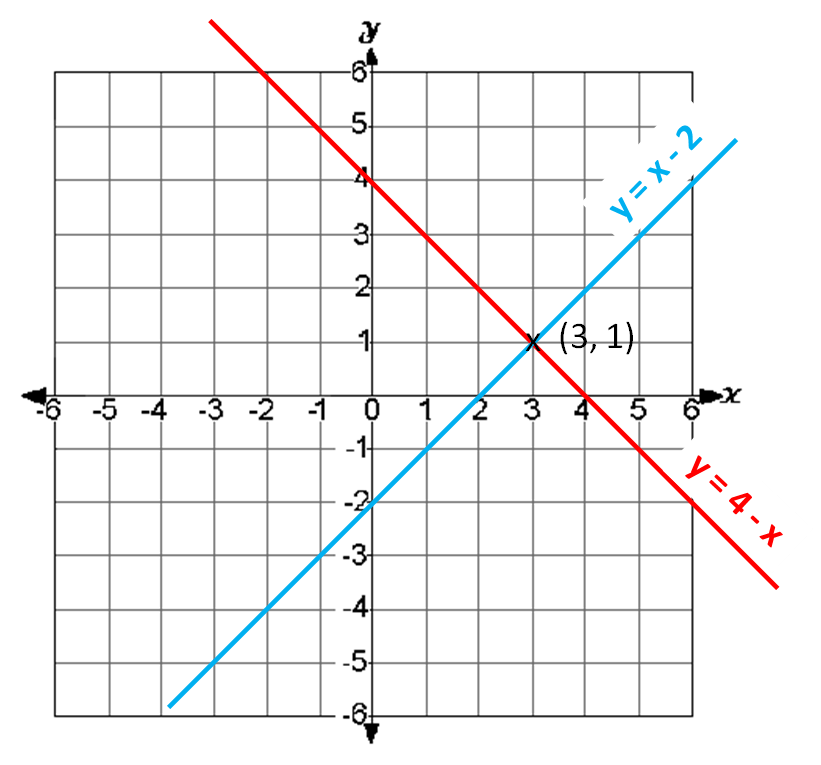The point of intersection is (3, 1).

• Question 2

By drawing the lines of these equations on a set of coordinate axes, solve the following equations simultaneously:

y = x - 2

y = 6 - x

x = 4, y = 2
EDDIE SAYS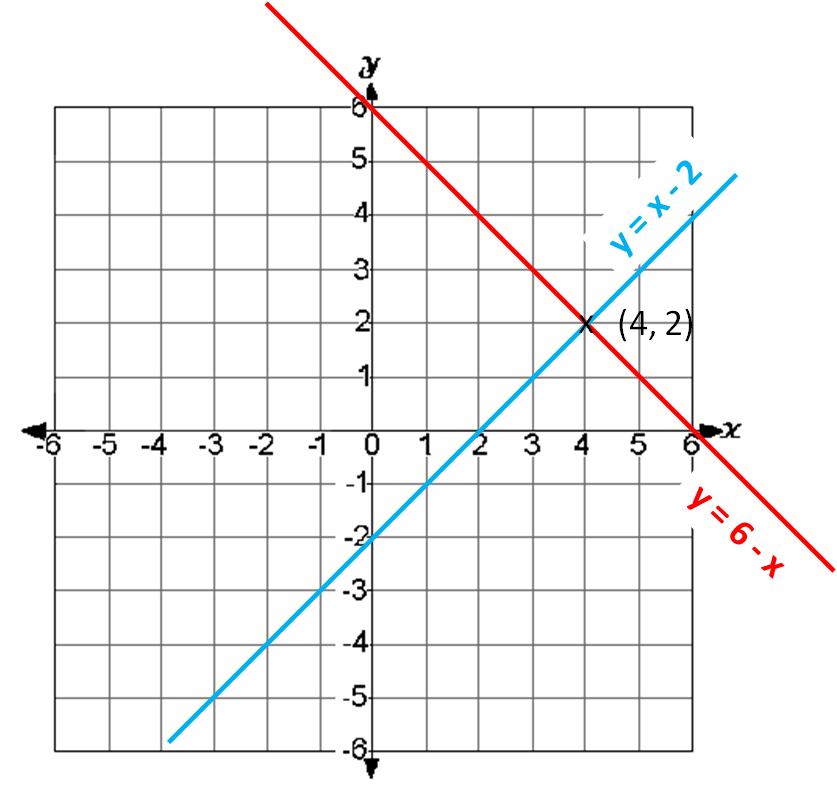The point of intersection is (4, 2).

• Question 3

By drawing the lines of these equations on a set of coordinate axes, solve the following equations simultaneously:

y = x - 2

y = 2 - x

x = 2, y = 0
EDDIE SAYSThe point of intersection is (2, 0).

• Question 4

By drawing the lines of these equations on a set of coordinate axes, solve the following equations simultaneously:

y = x - 2

y = -4 - x

x = -1, y = -3
EDDIE SAYS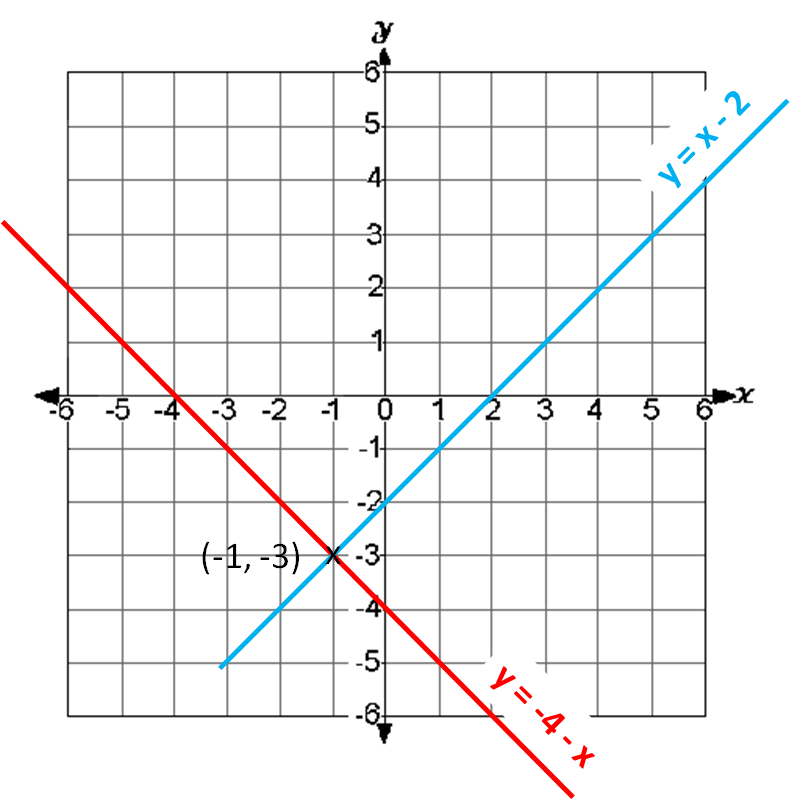The point of intersection is (-1, -3).

• Question 5

By drawing the lines of these equations on a set of coordinate axes, solve the following equations simultaneously:

y = x - 2

x + y = 0

x = 1, y = -1
EDDIE SAYSThe point of intersection is (1, -1).

• Question 6

By drawing the lines of these equations on a set of coordinate axes, solve the following equations simultaneously:

y = x + 6

x + y = 0

x = -3, y = 3
EDDIE SAYSThe point of intersection is (-3, 3).

• Question 7

By drawing the lines of these equations on a set of coordinate axes, solve the following equations simultaneously:

y = x + 6

x + y = -1

x = -3½, y = 2½
EDDIE SAYSThe point of intersection is (-3½, 2½).

• Question 8

By drawing the lines of these equations on a set of coordinate axes, solve the following equations simultaneously:

y = x - 3

x + y = -1

x = 1, y = -2
EDDIE SAYSThe point of intersection is (1, -2).

• Question 9

By drawing the lines of these equations on a set of coordinate axes, solve the following equations simultaneously:

y = x - 2

x + y = -3

x = -½, y = -2½
EDDIE SAYS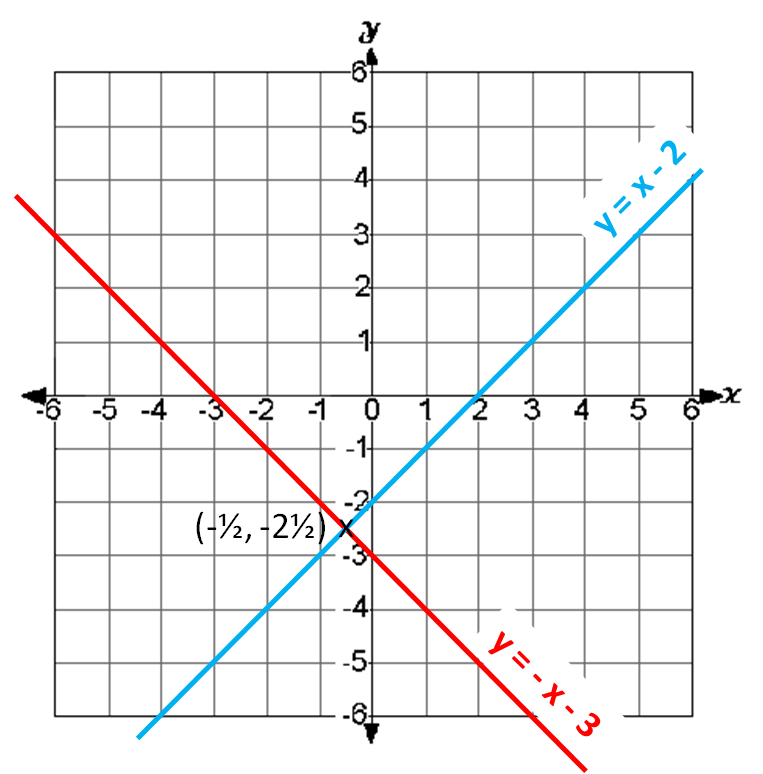The point of intersection is (-½, -2½).

• Question 10

By drawing the lines of these equations on a set of coordinate axes, solve the following equations simultaneously:

y - x = 5

y + x = 2

x = -1½, y = 3½
EDDIE SAYSThe point of intersection is (-1½, 3½).

---- OR ----

Sign up for a £1 trial so you can track and measure your child's progress on this activity.

### What is EdPlace?

We're your National Curriculum aligned online education content provider helping each child succeed in English, maths and science from year 1 to GCSE. With an EdPlace account you’ll be able to track and measure progress, helping each child achieve their best. We build confidence and attainment by personalising each child’s learning at a level that suits them.

Start your £1 trial